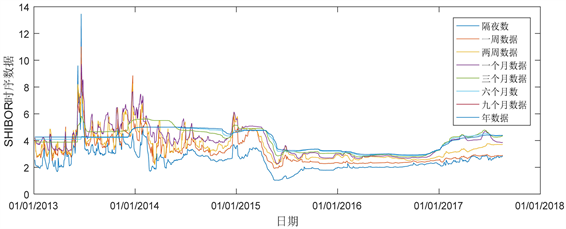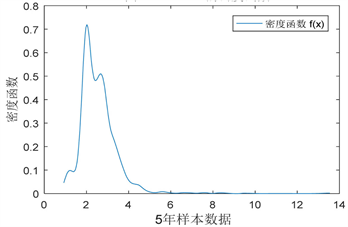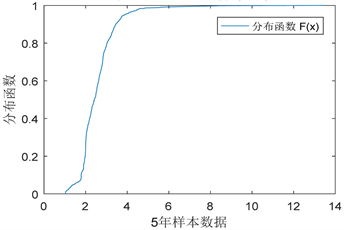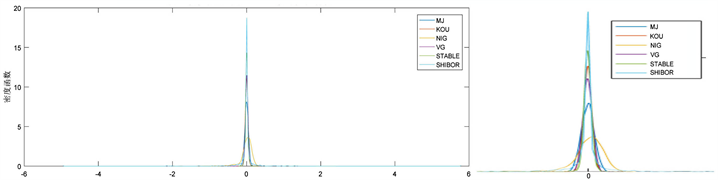SHIBOR时序数据分析：基于Levy过程模型Analyzing of SHIBOR Time Series: Based on Levy Process Models

• 全文下载: PDF(1268KB)    PP.255-264   DOI: 10.12677/FIN.2018.86030
• 下载量: 223  浏览量: 475

SHIBOR time series play an increasingly important role in the financial markets and related areas as the marketization of the RMB interest rate is more and more frequently advocated. Many scho-lars have made a number of studies on SHIBOR and the related fields. But most of them focus on the correlation between their own research fields and SHIBOR based upon regression model instead of on Levy process model and the characters of SHIBOR. This paper, from angles of real time and business time, by introducing Levy process, building multiple Levy process models and analyzing the results from the models, aims to reflect the changing trend and relevant characters of SHIBOR time series so as to enrich the research of SHIBOR time series and lay a sound foundation for the pricing of SHIBOR.

1. 引言

2. SHIBOR时间序列统计特征

SHIBOR时间序列有八个指标，分别为隔夜、一周、两周、一个月、三个月、六个月、九个月与年数据。为了对SHIBOR进行较好的研究，在统计分析上，我们采用了2013以来的数据，其时间序列路径如图1Figure 1. Path of SHIBOR time seriesTable 1. SHIBOR time series six major statistical indicatorsFigure 2. Density function of SHIBORFigure 3. The distribution function of SHIBOR

3. Levy过程模型

(一) Levy过程与Levy测度介绍Table 2. Measure and characteristic function of five kinds of Levy processes

(二) 具体模型介绍

${X}_{t}=\mu t+\sigma {W}_{t}+\underset{i=1}{\overset{{N}_{t}}{\sum }}{Y}_{i}$

$\text{E}\left[{\text{e}}^{iu{X}_{t}}\right]=\mathrm{exp}\left\{t\left(i\mu u-\frac{1}{2}{\sigma }^{2}{u}^{2}+\lambda \left({\text{e}}^{i\alpha u-\frac{1}{2}{\delta }^{2}{u}^{2}}-1\right)\right)\right\}$

$\text{E}\left[{\text{e}}^{iu{X}_{t}}\right]=\mathrm{exp}\left\{t\left[i\mu u-\frac{1}{2}{\sigma }^{2}{u}^{2}+iu\lambda \left(\frac{p}{{\eta }_{1}-iu}-\frac{1-p}{{\eta }_{2}+iu}\right)\right]\right\}$

$\text{E}\left[{\text{e}}^{iu{X}_{t}}\right]=\left\{\begin{array}{l}\mathrm{exp}\left\{t\left[i\mu u-\frac{1}{2}{\sigma }^{2}{u}^{2}-{\sigma }_{1}^{\alpha }{|u|}^{\alpha }\left(1-i\beta sign\left(u\right)\mathrm{tan}\frac{\text{π}\alpha }{2}\right)\right]\right\},\text{\hspace{0.17em}}\text{\hspace{0.17em}}\text{if}\text{\hspace{0.17em}}\alpha \ne 1\\ \mathrm{exp}\left\{t\left[i\mu u-\frac{1}{2}{\sigma }^{2}{u}^{2}-{\sigma }_{1}|u|\left(1+i\beta sign\left(u\right)\frac{2}{\text{π}}\mathrm{log}|\theta |\right)\right]\right\},\text{ }\text{ }\text{if}\text{\hspace{0.17em}}\alpha =1\end{array}$

4. 实证与检验

(一) 实证参数估计Table 3. Parameter estimation based on Merton jump process modelTable 4. Parameter estimation based on double exponential jump process modelTable 5. Parameter estimation based on normal inverse Gauss process modelTable 6. Parameter estimation based on variance gamma process modelTable 7. Parameter estimation based on α stable process

(二) 随机模拟Figure 4. Simulation path versus real path comparison

(三) 实证检验分析Figure 5. Analysis chart of Density function simulated sample and real SHIBOR sampleFigure 6. Analysis chart of distribution function of simulated sample and real SHIBOR sample

5. 结论

  蔡卫光, 张烨. 市场化条件下利率的决定因素——对我国银行同业拆借利率的协整分析[J]. 南开经济研究, 2005(6): 60-64  张丽娟. 我国银行间货币市场利率研究——兼论货币市场基准利率的选择[D]: [博士学位论文]. 上海: 复旦大学, 2007.  张一中. SHIBOR与中国的利率市场化[D]: [硕士学位论文]. 厦门: 厦门大学, 2009.  陈汉鹏, 戴金平. SHIBOR作为中国基准利率的可行性研究[J]. 管理世界, 2014(10): 37-46.  李海涛, 王欣, 方兆本. 基于偏t分布的SHIBOR隔夜拆借利率影响因素分析[J]. 系统工程, 2008, 26(9): 68-73.  潘松, 魏先华, 张敏, 宋洋, 陈敏. 银行间支付流与同业拆借利率之关系的实证研究[J]. 金融研究, 2009(1): 81-94.  陆伟伟. 我国SHIBOR影响因素的实证分析[J]. 财经论坛, 2017(9): 88-89.  何梦泽. 基于GARCH模型的SHIBOR波动性分析[J]. 统计与决策, 2013(11): 160-162.  高薇. 基于GARCH族模型的我国SHIBOR金融市场波动率统计研究[J]. 统计与决策, 2015(10): 30-33.  于建忠, 刘湘成. SHIBOR定价理论模型研究及其应用[J]. 金融研究, 2009(2): 40-53.  Heston, S.L. (1993) A Closed-Form Solution for Options with Stochastic Volatility with Applications to Bond and Currency Options. The Review of Financial Studies, 6, 327-343. https://doi.org/10.1093/rfs/6.2.327  Bates, D. (1996) Jumps and Stochastic Volatility: Exchange Rate Processes Implicit in Deutsche Mark Options. Review of Financial Studies, 9, 69-107. https://doi.org/10.1093/rfs/9.1.69  Merton, R.C. (1976) Option Pricing When Underlying Stock Returns Are Discontinuous. Journal of Financial Economics, 3, 125-144. https://doi.org/10.1016/0304-405X(76)90022-2  Chacko, G. and Viceira, L. (2003) Spectral GMM Estimation of Continuous-Time Processes. Journal of Econometrics, 116, 259-292. https://doi.org/10.1016/S0304-4076(03)00109-X  Hurst, S.R. and Platen, E. (1999) Option Pricing for a Log Stable Asset Price Model. Mathematical and Computer Modelling, 29, 105-119. https://doi.org/10.1016/S0895-7177(99)00096-5  Fang and Oosterlee (2008) A Novel Pricing Method for European Options Based on Fourier-Cosine Series Expansions.  Kou, S. (2002) Jump Diffusion Model for Option Pricing. Man-agement Science, 48, 1086-1101. https://doi.org/10.1287/mnsc.48.8.1086.166  Madan, D.B. and Seneta, E. (1987) Simulation of Estimation Using the Empirical Characteristic Function. International Statistics Review, 55, 153-161. https://doi.org/10.2307/1403191  Madan, D. and Seneta, E. (1990) The Variance Gamma Model for Share Market Returns. Journal of Business, 63, 511-524. https://doi.org/10.1086/296519  Weron, R. (1995) Performance of the Estimators of Stable Law Parameters. Applied Mathematics, No. 27, 1-20.  Nolan, J.P. (1998) Parameterizations and Modes of Stable Distributions. Statistics and Probability Letters, 38, 187-195. https://doi.org/10.1016/S0167-7152(98)00010-8